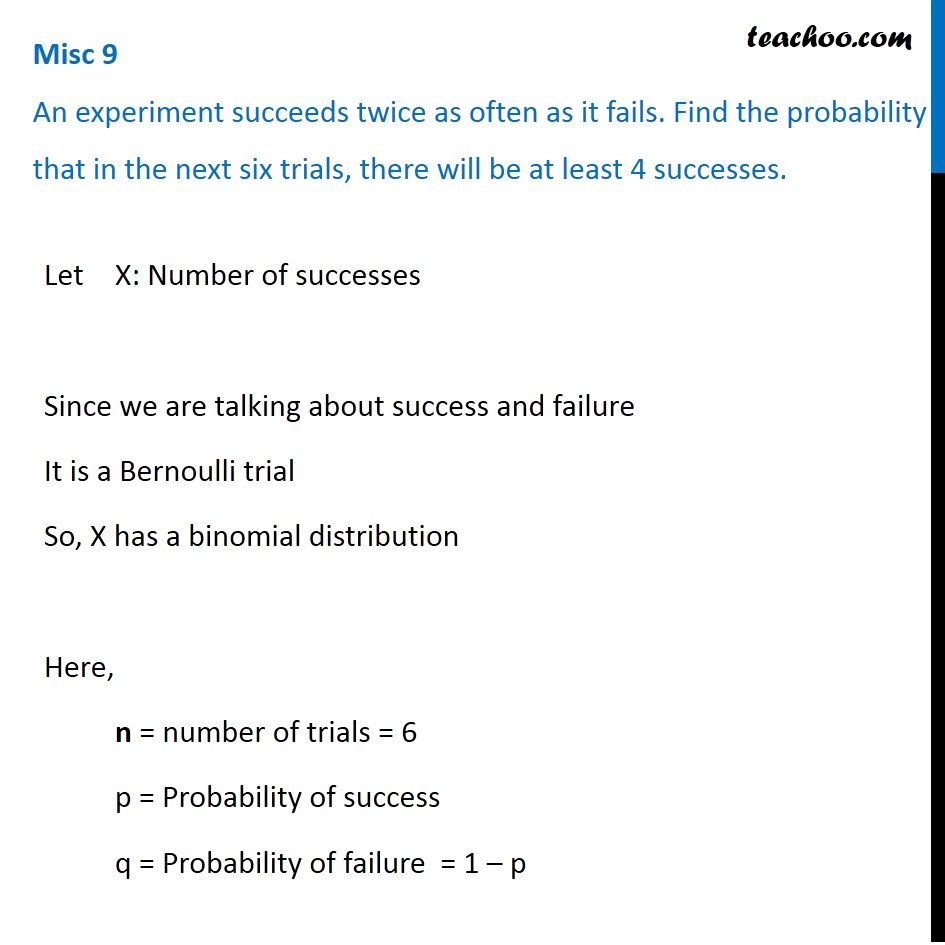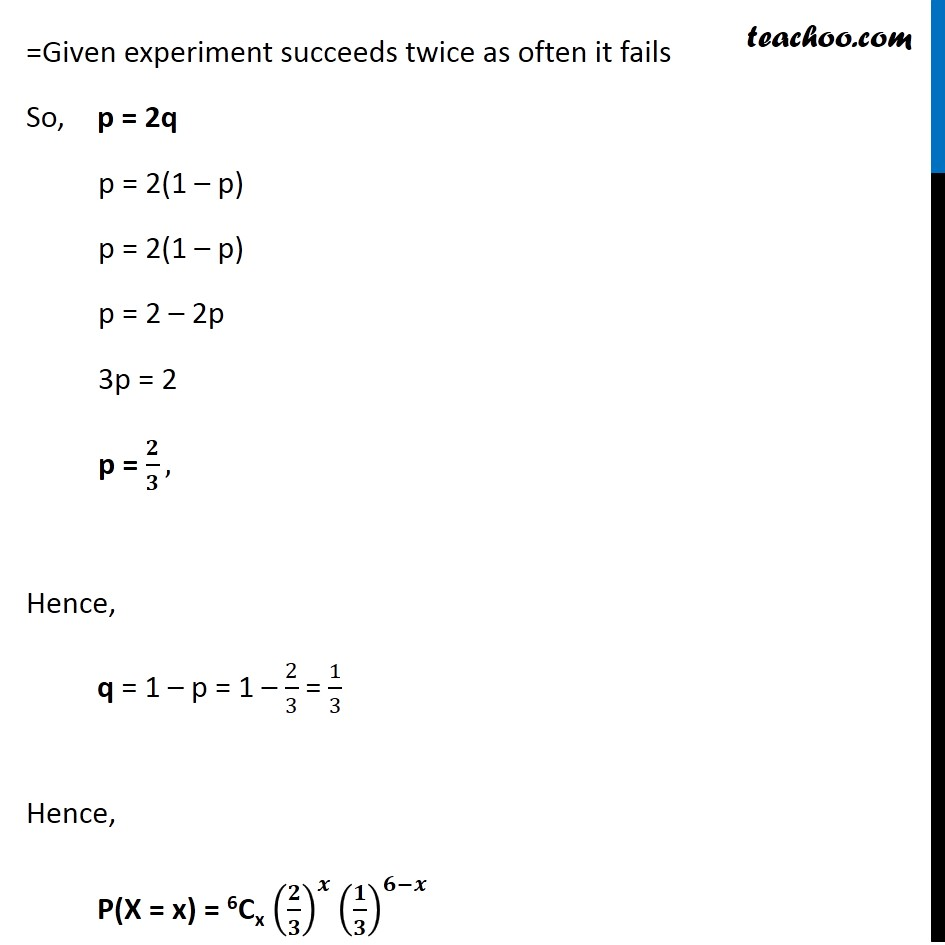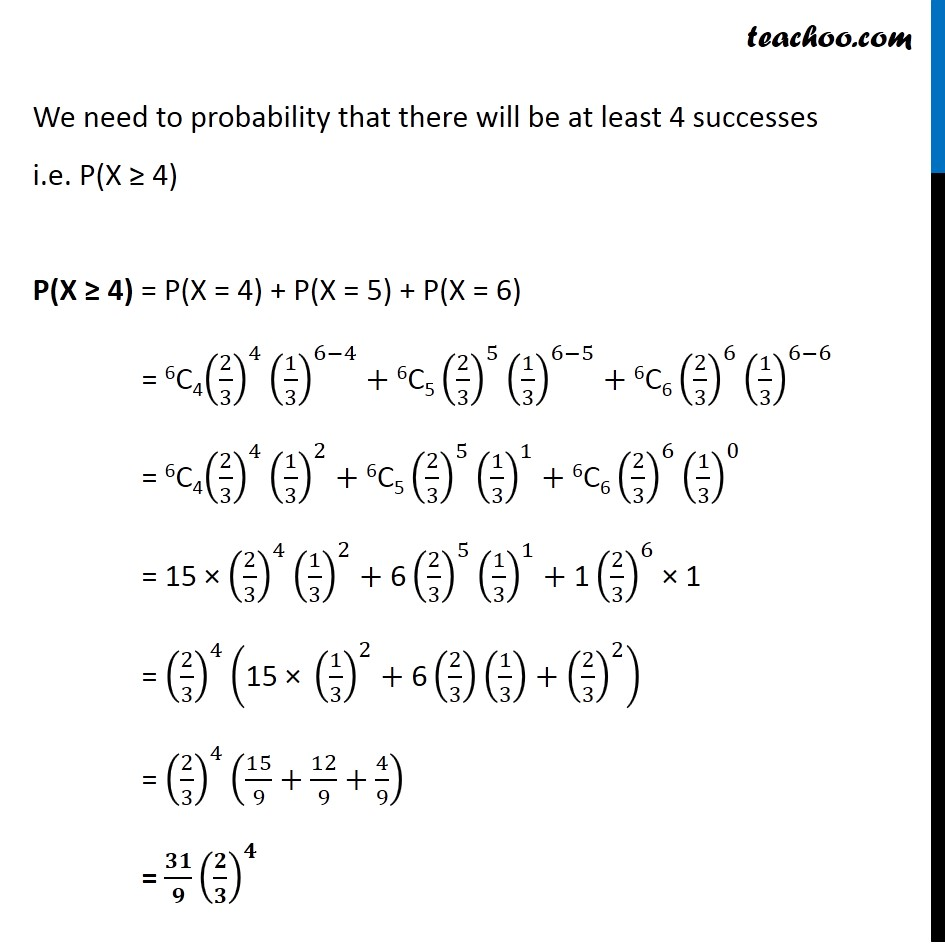Miscellaneous

Chapter 13 Class 12 Probability
Serial order wiseLearn in your speed, with individual attention - Teachoo Maths 1-on-1 Class

### Transcript

Question 4 An experiment succeeds twice as often as it fails. Find the probability that in the next six trials, there will be at least 4 successes. Let X: Number of successes Since we are talking about success and failure It is a Bernoulli trial So, X has a binomial distribution Here, n = number of trials = 6 p = Probability of success q = Probability of failure = 1 – p Let X: Number of successes Since we are talking about success and failure It is a Bernoulli trial So, X has a binomial distribution Here, n = number of trials = 6 p = Probability of success q = Probability of failure = 1 – p Let X: Number of successes Since we are talking about success and failure It is a Bernoulli trial So, X has a binomial distribution Here, n = number of trials = 6 p = Probability of success q = Probability of failure = 1 – p We need to probability that there will be at least 4 successes i.e. P(X ≥ 4) P(X ≥ 4) = P(X = 4) + P(X = 5) + P(X = 6) = 6C4(2/3)^4 (1/3)^(6−4)+"6C5" (2/3)^5 (1/3)^(6−5)+"6C6" (2/3)^6 (1/3)^(6−6) = 6C4(2/3)^4 (1/3)^2+"6C5" (2/3)^5 (1/3)^1+"6C6" (2/3)^6 (1/3)^0 = 15 × (2/3)^4 (1/3)^2+"6" (2/3)^5 (1/3)^1+"1" (2/3)^6 × 1 = (2/3)^4 ("15 × " (1/3)^2+"6" (2/3)(1/3)+(2/3)^2 ) = (2/3)^4 (15/9+12/9+4/9) = 𝟑𝟏/𝟗 (𝟐/𝟑)^𝟒